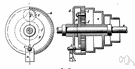# differential

(redirected from differential cell count)
Also found in: Thesaurus, Medical, Legal, Financial, Encyclopedia.

## dif·fer·en·tial

(dĭf′ə-rĕn′shəl)
adj.
1. Of, relating to, or showing a difference.
2. Constituting or making a difference; distinctive.
3. Dependent on or making use of a specific difference or distinction.
4. Mathematics Of or relating to differentiation.
5. Involving differences in speed or direction of motion.
n.
1. Mathematics
a. An infinitesimal increment in a variable.
b. The product of the derivative of a function of one variable and the increment of the independent variable.
2. A differential gear.
3. A difference between comparable things, as in wage rate or in price.

dif′fer·en′tial·ly adv.

## differential

(ˌdɪfəˈrɛnʃəl)
adj
1. of, relating to, or using a difference
2. constituting a difference; distinguishing
3. (Mathematics) maths of, containing, or involving one or more derivatives or differentials
4. (General Physics) physics engineering relating to, operating on, or based on the difference between two effects, motions, forces, etc: differential amplifier.
n
5. a factor that differentiates between two comparable things
6. (Mathematics) maths
a. an increment in a given function, expressed as the product of the derivative of that function and the corresponding increment in the independent variable
b. an increment in a given function of two or more variables, f(x1, x2, …xn), expressed as the sum of the products of each partial derivative and the increment in the corresponding variable
7. (Mechanical Engineering) an epicyclic gear train that permits two shafts to rotate at different speeds while being driven by a third shaft. See also differential gear
8. (Industrial Relations & HR Terms) chiefly Brit the difference between rates of pay for different types of labour, esp when forming a pay structure within an industry
9. (Commerce) (in commerce) a difference in rates, esp between comparable labour services or transportation routes
ˌdifferˈentially adv

## dif•fer•en•tial

(ˌdɪf əˈrɛn ʃəl)

adj.
1. of or pertaining to difference or diversity.
2. constituting a difference; distinguishing; distinctive.
3. exhibiting or depending upon a difference or distinction.
4. pertaining to or involving the difference of two or more motions, forces, etc.
5. pertaining to or involving a mathematical derivative or derivatives.
n.
6. a difference or the amount of difference, as in rate, cost, degree, or quality, between things that are comparable.
8. Math.
a. a function of two variables that is obtained from a given function, y = f(x), and that expresses the approximate increment in the given function as the derivative of the function times the increment in the independent variable, written as dy = f~(x) dx.
b. any generalization of this function to higher dimensions.
9. Physics. the quantitative difference between two or more forces, motions, etc.: a pressure differential.
[1640–50; < Medieval Latin]
dif`fer•en′tial•ly, adv.
ThesaurusAntonymsRelated WordsSynonymsLegend:
 Noun 1 differential - the result of mathematical differentiation; the instantaneous change of one quantity relative to another; df(x)/dxcurvature - the rate of change (at a point) of the angle between a curve and a tangent to the curvefiguring, reckoning, calculation, computation - problem solving that involves numbers or quantitiespartial, partial derivative - the derivative of a function of two or more variables with respect to a single variable while the other variables are considered to be constant 2 differential - a quality that differentiates between similar thingsdifference - the quality of being unlike or dissimilar; "there are many differences between jazz and rock" 3differential - a bevel gear that permits rotation of two shafts at different speeds; used on the rear axle of automobiles to allow wheels to rotate at different speeds on curvesdifferential gearbevel gear, pinion and crown wheel, pinion and ring gear - gears that mesh at an angle Adj. 1 differential - relating to or showing a difference; "differential treatment" 2 differential - involving or containing one or more derivatives; "differential equation"math, mathematics, maths - a science (or group of related sciences) dealing with the logic of quantity and shape and arrangement

## differential

noun
1. difference, gulf, gap, distinction, discrepancy, disparity, divergence, amount of difference Industrial wage differentials widened.
adjective
1. They may be forced to eliminate differential voting rights.
Translations
differentiaali

## differential

[ˌdɪfəˈrenʃəl]
A. ADJ [rate] → diferencial
B. N
1. (esp Brit) (Econ) →
see also wage
2. (Math) →
C. CPD differential calculus N
differential equation N

## differential

[ˌdɪfəˈrɛnʃəl] n
(= difference) →
(AUTOMOBILES) [car] → differential calculus n

## differential

adj (= different) rates of pay, treatment, diagnosis, effectsunterschiedlich; (= distinguishing) featureunterscheidend; differential pricing (Comm) → Preisdiskriminierung f
n
(= difference)Unterschied m(between zwischen); (Math) → Differenzial nt, → Differential nt; wage/salary differentialLohn-/Gehaltsunterschiede or -differenzen pl
(Aut) → Differenzial(getriebe) nt, → Differential(getriebe) nt

## differential

:
differential calculus
differential coefficient
n (Math) → Ableitung f
differential equations
differential gear
n (Aut) → Differenzial(getriebe) nt, → Differential(getriebe) nt

## differential

[ˌdɪfəˈrɛnʃl]
1. n (Econ) → (Math, Aut) →
2. adjdifferenziale
differential calculus (Math) → calcolo differenziale
differential erosion →

## dif·fer·en·tial

a. diferencial, rel. a la diferenciación;
___ diagnosisdiagnóstico ___.
References in periodicals archive ?
Cytological analysis comprises of microscopic examination, wherein the total and differential cell count is performed using a haemocytometer.
To perform differential cell count, cellular slime was smeared onto slides using a cytospin (500 r/min for 5 min) and air-dried.
Hence, the first objective of this study was to investigate aspects of the cellular response of the zebra mussel (total hemocyte count [THC], differential cell count, and phagocytosis) as well as some humoral factors (e.
Analysis of pleural fluid aspirates should include the following: Basic * Stains for AFB, TB culture * Bacterial MCS * Cytology * Differential cell count * ADA * LDH * Protein Additional * Albumin * Glucose * IFN-[gamma] * Fungal culture * Lipid analysis * Haematocrit * Amylase * Tumour markers * Complement C4 level
Rou-tine laboratory measurements, including complete blood count, differential cell count, erythrocyte sedimentation rate, liver and renal function tests, urinalysis, and serum calcium levels were normal.

Site: Follow: Share:
Open / Close